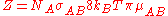xCollision frequencyEncyclopedia
Collision frequency is defined in chemical kinetics
Chemical kinetics
Chemical kinetics, also known as reaction kinetics, is the study of rates of chemical processes. Chemical kinetics includes investigations of how different experimental conditions can influence the speed of a chemical reaction and yield information about the reaction's mechanism and transition...

, in the background of theoretical kinetics, as the average number of collisions between reacting molecules per unit of time. Its symbol is Z.
For a gas phase bimolecular reaction Z is• NA is the number of atoms B per unit volume (m^3)
• σAB is the reaction cross section
Cross section (physics)
A cross section is the effective area which governs the probability of some scattering or absorption event. Together with particle density and path length, it can be used to predict the total scattering probability via the Beer-Lambert law....

• kB is Boltzmann constant
• μaB is the reduced mass
Reduced mass
Reduced mass is the "effective" inertial mass appearing in the two-body problem of Newtonian mechanics. This is a quantity with the unit of mass, which allows the two-body problem to be solved as if it were a one-body problem. Note however that the mass determining the gravitational force is not...

of the reactants